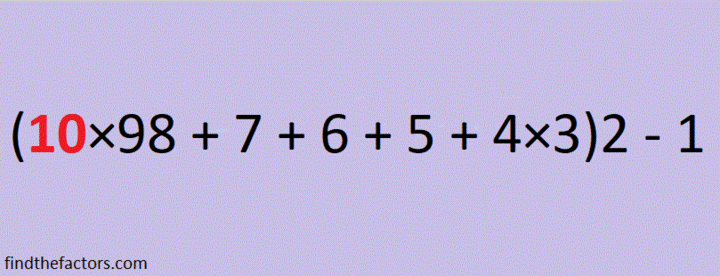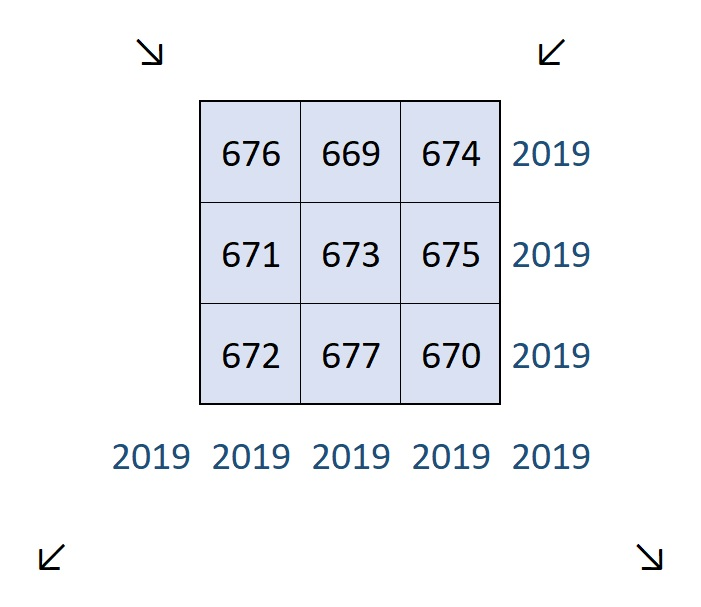# Facts about and Factors of 2019

Here’s a countdown you can use to ring in the New Year:make science GIFs like this at MakeaGif

2019 is the sum of consecutive numbers three different ways:
1009 + 1010 = 2019
672 + 673 + 674 = 2019
334 + 335 + 336 + 337 + 338 + 339 = 2019

There is one way that 2019 is the sum of consecutive odd numbers:
671 + 673 + 675 = 2019

2019 is the difference of two squares two different ways:
338² – 335² = 2019
1010² – 1009² = 2019

2019 is the sum of three squares nine different ways:
43² + 13² + 1² = 2019
43² + 11² + 7² = 2019
41² + 17² + 7² = 2019
41² + 13² + 13² = 2019
37² + 25² + 5² = 2019
37² + 23² + 11² = 2019
37² + 19² + 17² = 2019
35² + 25² + 13² = 2019
31² + 23² + 23² = 2019

2019 is the hypotenuse of a Pythagorean triple:
1155-1656-2019 so 1155² + 1656² = 2019²

2¹⁰ + 2⁹ + 2⁸ + 2⁷ + 2⁶ + 2⁵ + 2¹ + 2⁰ = 2019

2019 is a palindrome in a couple of bases:
It’s 5B5 in BASE 19 (B is 11 base 10) because 5(19²) + 11(19) + 5(1) = 2019,
and 3C3 in BASE 24 (C is 12 base 10) because 3(24²) + 12(24) + 3(1) = 2019

Every year has factors that often catch people by surprise. Today I would like to give you my predictions for the factors of 2019:
2019 will have four positive factors: 1, 3, 673, and 2019
However, 2019 will also have four negative factors: -1, -3, -673, and -2019

Which factors, positive or negative, will be your focus in the coming year?Finally, I’ll share some mathematics-related 2019 and New Year tweets that I’ve seen on twitter. Some of these tweets have links that contain even more facts about the number 2019.

That tweet inspired me to make my own 3 × 3 Magic Square where every number is different but every row, column and diagonal totals the same number:But because 2019 is divisible by 3, it can also be a magic sum of 3 × 3 magic square:5

5

And finally, here is my contribution to 2019 twitter:

## 2 thoughts on “Facts about and Factors of 2019”

1.Edmark M. Law

2019 = (1 + 2) × 3 + (45 + 67 + 89) × 10
= 10 × (−9 + (8 + 7) × (−6 + 5 × 4)) + 3 × (2 + 1)
= 0³ + 1⁸ + 2⁷ – 3⁹ + 4⁶ + 5⁴ + 6² + 7⁵ + 8¹ + 9⁰ (pandigital)
= 16 + 442 + 750 + 811 = 1⁶ + 44² + 75⁰ + 81¹

•ivasallay

Thank you for these and all the other ones you put on your website!

This site uses Akismet to reduce spam. Learn how your comment data is processed.